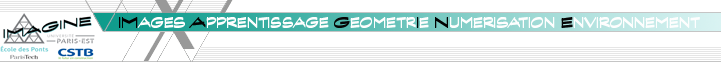Home Members Research Teaching Demos Software

Research
 Domain : 3D Learning Vision Constraint Prog. Sustain. Devel. Medical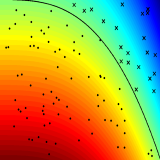Statistical learning [ALT11] Competing against the best nearest neighbor filter in regression [COLT11b] Tight conditions for consistent variable selection in high dimensional nonparametric regression [COLT11a] Optimal aggregation of affine estimators [AOS11] [AIHP11] Second-order asymptotic expansion for a non-synchronous covariation estimator [HAL09a] Risk bounds in linear regression through PAC-Bayesian truncation [AoS08] Fast learning rates in statistical inference through aggregation [NIPS07] Progressive mixture rules are deviation suboptimal [CERTIS0735] No fast exponential deviation inequalities for the progressive mixture rule [AS06] Fast learning rates for plug-in classifiers [JMLR07a] Combining PAC-Bayesian and generic chaining bounds [NIPS06w] Use of variance estimation in the multi-armed bandit problem [CERTIS0620] Fast learning rates in statistical inference through aggregation [COLT06] A randomized online learning algorithm for better variance control [PP05] Fast learning rates for plug-in estimators under the margin condition [B04] Aggregated estimators and empirical complexity for least square regression [PhD04a] PAC-Bayesian Statistical Learning Theory [NIPS04] PAC-Bayesian Generic Chaining [PP0401] A better variance control for PAC-Bayesian classification [PP0402] Classification under polynomial entropy and margin assumptions and randomized estimators [PP03] Aggregated estimators and empirical complexity for least square regressionGoodness-of-fit testing [JMLR12] Minimax hypothesis testing for curve registration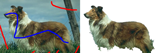Image Segmentation [JCMA11] Description of random fields by means of one-point finite-conditional distributions [ACCV09a] Transductive segmentation of textured meshes [CVPR08a] Segmentation by transduction [SSVM07] Towards segmentation based on a shape prior manifold [CERTIS0627] Transductive segmentation [CERTIS0626] Fast interactive segmentation using color and textural information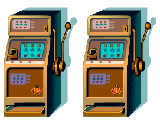Exploration vs Exploitation [ALT11a] Deviations of Stochastic Bandit Regret [COLT11 ] Minimax policies for combinatorial prediction games [HDR10] PAC-Bayesian aggregation and multi-armed bandits [JMLR10] Regret Bounds and Minimax Policies under Partial Monitoring [COLT10] Best Arm Identification in Multi-Armed Bandits [AISTATS10] Regret bounds for Gaussian process bandit problems [book_chapter] Bandit view on noisy optimization [COLT09a] Minimax policies for adversarial and stochastic bandits [NIPS08] Algorithms for Infinitely Many-Armed Bandits [ICML08b] Empirical Bernstein stopping [TCS08] Exploration-exploitation trade-off using variance estimates in multi-armed bandits [ALT07] Tuning bandit algorithms in stochastic environments [CERTIS0731] Variance estimates and exploration function in multi-armed bandit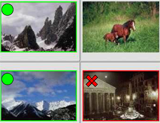Image Retrieval [PR11] Semantic hierarchies for image annotation: a survey [ECCV2010b] Towards Optimal Naive Bayes Nearest Neighbor [PhD10] Hiérarchies sémantiques pour lannotation multifacette dimages [MIR08] Semantic Lattices for Multiple Annotation of Images [CBMI08] Object Recognition and Retrieval by Context Dependent Similarity Kernels [CVPR08b] Manifold Learning using Robust Graph Laplacian for Interactive Image Retrieval [ICASSP08] Interactive Image Retrieval [TSI0718] Context-Dependent Kernel Design for Object Matching and Recognition [ISNN07] A Particular Gaussian Mixture Model for Clustering [CERTIS0732] Graph Laplacian for Interactive Image Retrieval [CERTIS0730] Graph-cut transducers for relevance feedback in content based image retrievalSparse recovery [HAL09b] Structured Variable Selection with Sparsity-Inducing Norms [MLVMA09] Sparse Learning Approach to the Problem of Robust Estimation of Camera Locations [COLT09b] Sparse Regression Learning by Aggregation and Langevin Monte-Carlo [ML08a] Aggregation by exponential weighting, sharp oracle inequalities and sparsity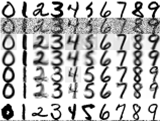Manifold learning and denoising [ICIP08] Normalization and Preimage Problem in Gaussian Kernel PCA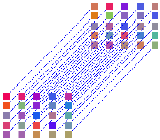Kernel Methods [ICML08a] Robust Matching and Recognition using Context-Dependent Kernels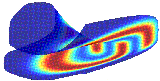Manifold learning [JMLR08a] A new algorithm for estimating the effective dimension-reduction subspace [NIPS07w] Toward Manifold-Adaptive Learning [ICML07] Manifold-adaptive dimension estimation [JMLR07b] Graph laplacians and their convergence on random neighborhood graphs [ICML05] Intrinsic dimensionality estimation of submanifolds in R^d [COLT05] From graphs to manifolds - weak and strong pointwise consistency of graph Laplacians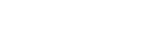Machine and speech translation [ICASSP07] Consensus Network Decoding For Statistical Machine Translation System Combination